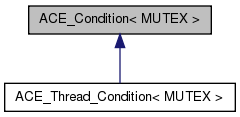ACE 6.0.1

# ACE_Condition< MUTEX > Class Template Reference

ACE_Condition variable wrapper, which allows threads to block until shared data changes state. More...

`#include <Condition_T.h>`

Inheritance diagram for ACE_Condition< MUTEX >:[legend]

List of all members.

## Public Member Functions

ACE_Condition (MUTEX &m, int type=USYNC_THREAD, const ACE_TCHAR *name=0, void *arg=0)
Initialize the condition variable.
~ACE_Condition (void)
Implicitly destroy the condition variable.
int wait (const ACE_Time_Value *abstime)
int wait (void)
Block on condition.
int wait (MUTEX &mutex, const ACE_Time_Value *abstime=0)
int signal (void)
int remove (void)
Explicitly destroy the condition variable.
MUTEX & mutex (void)
Returns a reference to the underlying mutex_;.
void dump (void) const
Dump the state of an object.

## Protected Attributes

ACE_cond_t cond_
Condition variable.
MUTEX & mutex_
Reference to mutex lock.

## Private Member Functions

void operator= (const ACE_Condition< MUTEX > &)
ACE_Condition (const ACE_Condition< MUTEX > &)

## Detailed Description

### template<class MUTEX> class ACE_Condition< MUTEX >

ACE_Condition variable wrapper, which allows threads to block until shared data changes state.

A condition variable enables threads to atomically block and test the condition under the protection of a mutual exclu- sion lock (mutex) until the condition is satisfied. That is, the mutex must have been held by the thread before calling wait or signal on the condition. If the condition is false, a thread blocks on a condition variable and atomically releases the mutex that is waiting for the condition to change. If another thread changes the condition, it may wake up waiting threads by signaling the associated condition variable. The waiting threads, upon awakening, reacquire the mutex and re-evaluate the condition. Note, you can only parameterize <ACE_Condition> with ACE_Thread_Mutex, ACE_Recursive_Thread_Mutex, or ACE_Null_Mutex.

## Constructor & Destructor Documentation

template<class MUTEX >
 ACE_Condition< MUTEX >::ACE_Condition ( MUTEX & m, int type = `USYNC_THREAD`, const ACE_TCHAR * name = `0`, void * arg = `0` )

Initialize the condition variable.

template<class MUTEX >
 ACE_Condition< MUTEX >::~ACE_Condition ( void )

Implicitly destroy the condition variable.

template<class MUTEX >
 ACE_Condition< MUTEX >::ACE_Condition ( const ACE_Condition< MUTEX > & ) ` [private]`

## Member Function Documentation

template<class MUTEX >
 int ACE_Condition< MUTEX >::broadcast ( void ) ` [inline]`

template<class MUTEX >
 void ACE_Condition< MUTEX >::dump ( void ) const

Dump the state of an object.

template<class MUTEX >
 MUTEX & ACE_Condition< MUTEX >::mutex ( void ) ` [inline]`

Returns a reference to the underlying mutex_;.

template<class MUTEX >
 void ACE_Condition< MUTEX >::operator= ( const ACE_Condition< MUTEX > & ) ` [private]`
template<class MUTEX >
 int ACE_Condition< MUTEX >::remove ( void ) ` [inline]`

Explicitly destroy the condition variable.

template<class MUTEX >
 int ACE_Condition< MUTEX >::signal ( void ) ` [inline]`

template<class MUTEX >
 int ACE_Condition< MUTEX >::wait ( void )

Block on condition.

template<class MUTEX >
 int ACE_Condition< MUTEX >::wait ( MUTEX & mutex, const ACE_Time_Value * abstime = `0` )

Block on condition or until absolute time-of-day has passed. If abstime == 0 use "blocking" wait() semantics on the <mutex> passed as a parameter (this is useful if you need to store the <Condition> in shared memory). Else, if <abstime> != 0 and the call times out before the condition is signaled <wait> returns -1 and sets errno to ETIME.

template<class MUTEX >
 int ACE_Condition< MUTEX >::wait ( const ACE_Time_Value * abstime )

Block on condition, or until absolute time-of-day has passed. If abstime == 0 use "blocking" <wait> semantics. Else, if abstime != 0 and the call times out before the condition is signaled <wait> returns -1 and sets errno to ETIME.

## Member Data Documentation

template<class MUTEX >
 ACE_cond_t ACE_Condition< MUTEX >::cond_` [protected]`

Condition variable.

template<class MUTEX >
 MUTEX& ACE_Condition< MUTEX >::mutex_` [protected]`

Reference to mutex lock.

The documentation for this class was generated from the following files: